Introduction >
The structural frame
The construction
The reinforcement I
The reinforcement II
Quantity/Cost estimation
Detailing drawingsIntroduction >
Wind and Seismic Forces >
Structural model and Analysis
Modelling slabs
Slabs
Seismic behavour of frames
Appendix A
Appendix B
Appendix C
Appendix DIntroduction >
Materials
To be continued >

## x horizontal component of the seismic action: Calculation of seismic forces using CQC for all mode shapes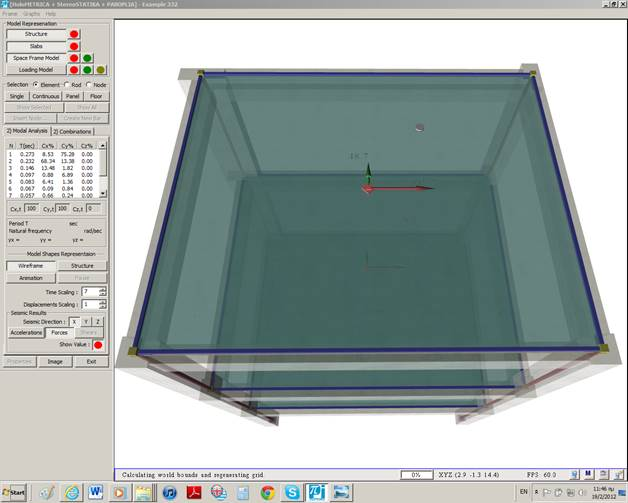HoloBIM uses CQC to all stress resultants and displacements. All stress resultants are examined for both positive and negative signs (32 combinations), thus the results are satisfactory.

## Seismic accelerations for x and y horizontal components of the seismic action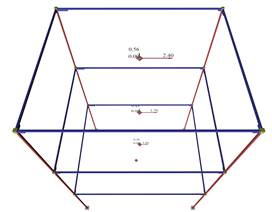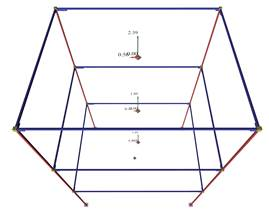HoloBIM uses also another simple method, overcoming the sign related problem providing reasonable results according to classic structural analysis (see § of Seismic accelerations). The steps of the method are:
· The calculation of seismic accelerations ai,j (j=x,y,z), at any point i along X, Y, Z directions, using CQC. The calculation takes into account the soil parameters of the earthquake, the seismic ground acceleration, the masses participating at the time of the earthquake at the point i and the available ductility of the building. The sign accelerations ai,j derives from the dominant mode (i.e. the mode with the highest modal participation factor).
· The calculation of seismic forces Hi,j=ai,j×Mi at any point i, as the algebraic product of the seismic acceleration and the mass at this point.
· The calculation of seismic stresses in X, Y, Z direction for every seismic combination (e.g. earthquake in Y + 30%, earthquake in X + 20%, earthquake in Z), based of the seismic forces.
· In case the seismic combination includes accidental eccentricities, each mass M of a node i is placed at the position of the node i offset by the signed value (+ or –) of the eccentricities. In this way, all cases of nodes are resolved, whether they belong to the diaphragm or not. If all nodes of a floor belong to the diaphragm, the resultant loading is equivalent to the one that would result if the total mass of the floor was offsetted from the center of mass by the amount of the accidental eccentricities.
· Τhe stress resultants of each combination are signed according to the classic structural analysis.

## Ten-storey building of a non-symmetrical dual system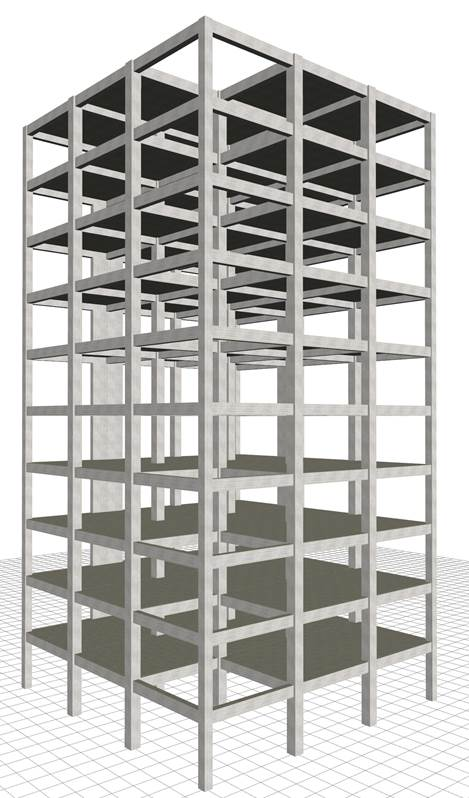(project <b_547-3b>)

## y horizontal component of the seismic action: Distribution of seismic accelerations – forces - shears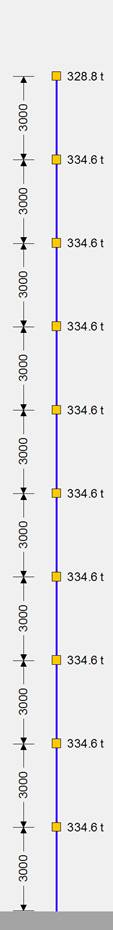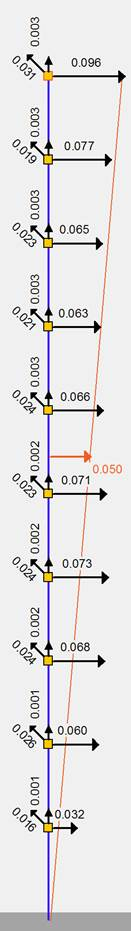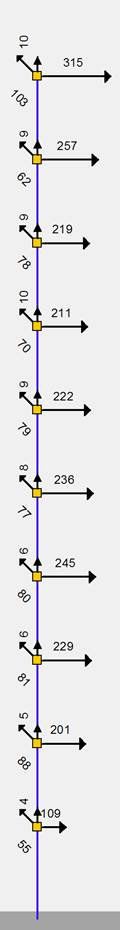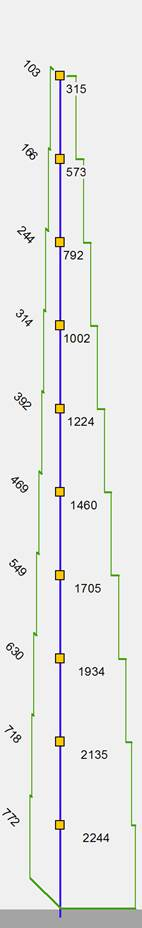Τhe advantage of the second method is that it provides orders of magnitudes in terms of classic structural analysis understandable to the engineer. For instance, the seismic accelerations at the diaphragmatic floors of common buildings should follow an almost triangular distribution with zero value at the base of the building and the design acceleration ao at its centroid. Τhis triangle is indicated by the red dashed line at the Interface of the related software so that the engineer is able to check the order of magnitude of the distribution of the seismic accelerations.